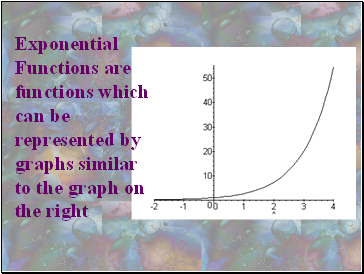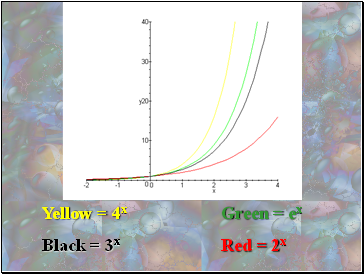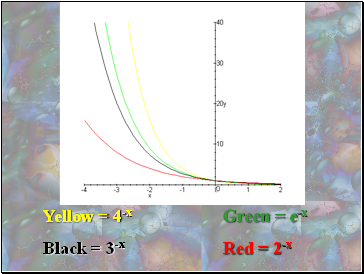# Exponential functionsPage 1

#### WATCH ALL SLIDES

Slide 1## Exponential functions

Slide 2Exponential Functions are functions which can be represented by graphs similar to the graph on the right

Slide 3All base exponential functions are similar because they all go through the point (0,1), regardless of the size of their base number

Exponential Functions are written in the form:

y = abx

a= constant b = base x = variable

Slide 4When solving exponential equations, it is important to have both sides of the equation set to the same base

When working with exponential equations, the Laws of Exponents still hold true

Slide 5Yellow = 4x Green = ex

Black = 3x Red = 2x

Slide 6As you could see in the graph, the larger the base, the faster the function increased

If we place a negative sign in front of the x, the graphs will be reflected(flipped) across the y-axis

Slide 7Yellow = 4-x Green = e-x

Black = 3-x Red = 2-x

Slide 8By using Microsoft Excel, we can make a table of values and a graph of the data

y = ex

Slide 9We can also use Maple 7 to plot exponential graphs

> plot (exp(x),x=-5 5);